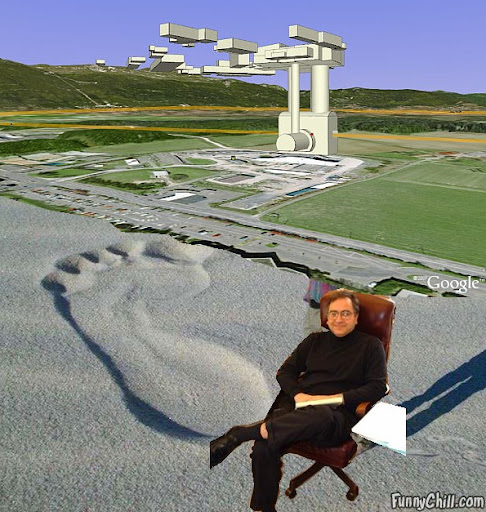## Wednesday, May 19, 2010

### Fuzzy F-theory

Mark Van Raamsdonk has a new preprint on the entanglement glue,
Building up spacetime with quantum entanglement
and I think it is deep and at least morally true. But because I have already written about it in July 2009, let me discuss another paper that is at least comparably interesting.Both Jonathan Heckman and Herman Verlinde have written papers about the bottom-up string phenomenology. Verlinde would work with D-branes while Heckman was focusing on F-theory. Now they teamed up to find something exciting about the F-theory model building:
Evidence for F(uzz) theory
Imagine a GUT 7-brane in a local F-theory phenomenological model. Its 4 real dimensions are typically compactified on a del Pezzo-like cycle inside the compact geometry while the remaining 3+1 dimensions coincide with the world we know and love.

What happens if the del Pezzo volume shrinks to zero size? Is there a new alternative description? The authors argue that the integral of "(B+F) ∧ (B+F)" over the del Pezzo surface has to be quantized (up to the right factors of "2π" etc.) - essentially integer, N.

Such a condition typically means that the world volume also becomes non-commutative: the commutator of two coordinates is a c-number (in the flat limit). It's the case here, too. The del Pezzo surface becomes a fuzzy geometry which is de facto composed out of N points only. You should imagine that the Kaluza-Klein tower over the del Pezzo surface gets truncated, much like in deconstruction, but the algebraic properties of the continuous geometry are more peacefully preserved.

The 7-branes may then also be visualized as a composite of N D3-branes - or 5N D3-branes because the SU(5) gauge group increases the multiplicity in a similar way as the points on the fuzzy space.

Moreover, the GUT gauge coupling is not arbitrary! Because this whole model is embedded in F-theory, the coupling and its S-dual value are related by SL(2,Z) transformations etc. In particular, the F-theory models have to include a point where the complex dilaton-axion coupling of type IIB string theory equals the third root of unity: that's the point where the gauge group gets enhanced to an E6 and such a point has to exist.

This constraint turns out to imply that the GUT gauge coupling is not arbitrary: the inverse "GUT fine structure constant", 1/α_{GUT}, has to be equal to N. (There is some probably calculable additional coefficient here similar to sqrt(3/4) or something like that which the authors don't quite unmask, for reasons that are unknown to me.)

So when you hear that the GUT coupling has to be α = 1/24 at the GUT scale, such a formula could actually be exact - or 1/25 may be the exact value. The possible values of the coupling are restricted to a discrete set. Moreover, each allowed value restricts the spectrum of the theory, too. To learn something about these conditions, Verlinde and Heckman study the fuzzy versions of concepts such as the Landau-Ginzburg model, the flop transitions, intersection rules, and many other things.

At any rate, this F-theory framework seems to be extraordinarily predictive. At the end, the low-energy couplings of the allowed F-theory models can cover a big portion of the parameter space that is allowed in the effective field theories. However, the density of the allowed points can be pretty low and in a realistic neighborhood of an effective field theory, the allowed string vacuum may be essentially unique.

All these insights are "new physics" that wouldn't be discovered if one adopted the anthropic attitude that "anything goes". It's not true that anything goes and there are always important constraints on the possible phenomena and properties of objects - constraints that we should be learning increasingly well because this is what physics is really about.